# Reachable Sets for Some Simple Models of the Car's Motion

## V.L.Turova

### All slides in PDF (2983 Kb)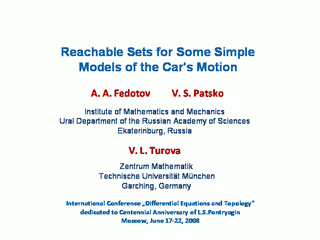## Mathematical cars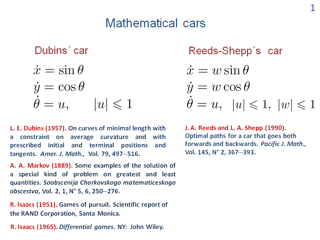The talk is devoted to reachable sets for two very simple models of the car's dynamics. We call them ''mathematical cars''.

The simplest model is to the left. The name "Dubins' car" is related to the paper by L. E. Dubins. In this paper, the theorem on the number and type of optimal control switches was proved for time-optimal problems with such dynamics. An identical description of dynamics was used by R. Isaacs and, more than one hundred years ago, by A. A. Markov.

The difference of the second model is in the presence of some additional control w. By means of it, we can change the magnitude of the linear velocity instantaneously. For w equal to one, the car moves forward, for w equal to minus one, it goes backwards. The time-optimal control problem for such a model was investigated in the paper by J. A. Reeds and L. A. Shepp.

## Given-time reachable sets in the plane of geometric coordinates (Dubins' car)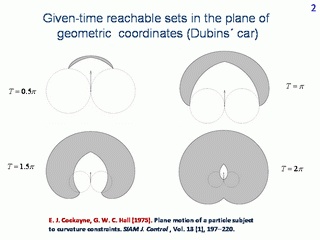Let us fix the geometrical state and direction of the forward motion at the initial instant. For Dubins' car, the forward motion direction coincides with the velocity direction. On the slide, the form of reachable sets at given instants is shown. These are sets of points which are reachable from the initial state at a given time. We will call them the given-time reachable sets. The structure of the given-time reachable sets for Dubins' car is investigated in the paper by E. J. Cockayne and G. W. C. Hall.

## Time-limited reachable sets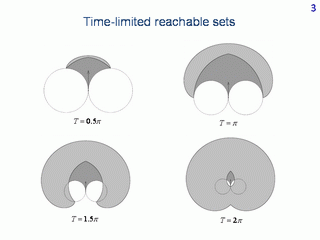Similar reachable sets but by a given time are depicted. These are sets of points which are reachable from the initial state within a given time. We call them the time-limited reachable sets. More dark colouring is related to the parts which show the difference comparing to the given-time reachable sets.

## Given-time reachable sets (Reeds-Shepp's car)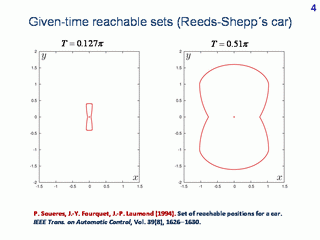For Reeds-Shepp's car, given-time reachable sets are symmetrical with respect to both vertical and horizontal axes. Moreover, they grow monotonically with time, and, therefore, coincide with corresponding time-limited reachable sets. Due to these facts, Reeds-Shepp's model is so popular in papers on theoretical robotics. The structure of reachable sets for Reeds-Shepp's car was investigated in the paper by P. Soueres, J.-Y. Fourquet, J.-P. Laumond.

## From Dubins' car to Reeds-Shepp's car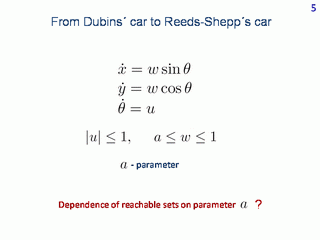Let us introduce a parameter "a" which determines the lower bound on the control w. If a > 0, the forward motion is only possible. If a < 0, the car can additionally move backwards. For a = 1, one obtains Dubins' car, for a = -1, Reeds-Shepp's car occurs.

## Isaacs' transformation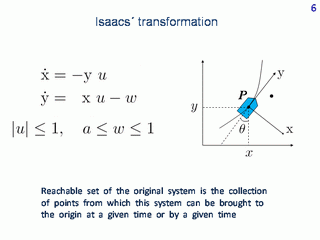To construct numerically reachable sets in the plane of geometrical coordinates, let us apply Isaacs' transformation. We put the origin of the reference sytem at the position of object P. Let axis y be directed along the forward motion direction. Let us fix some point in the original plane. Then, in the reference coordinates, the motion of the point is described by the system to the left.

The time-limited or given-time reachable set in the plane of original coordinates coincides with the set of all reference points from which the origin of the reference system can be reached within a given or at a given time. The latter set will be called the solvability set.

## Backward construction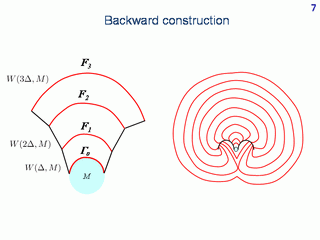To construct given-time or time-limited solvability sets in the reference coordinates, we apply backward constructions. They are schematically shown here for the time-optimal problem. We move backwards in time with time step Δ. The target set not necessarily coincides with the origin and is not necessarily a single point. It can be any convex set in the plane.

## Given-time reachable sets for different values of a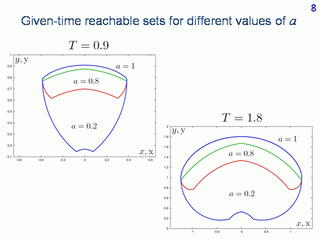Here, the given-time reachable sets are shown for two instants. The computation is done for three values of parameter a. The target set in the backward computation is the origin of the reference system.

## Time-limited reachable sets for different values of a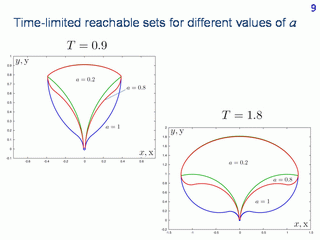Here, similar pictures of the time-limited reachable sets are shown.

## Reachable sets corrected by accounting for oriented added shift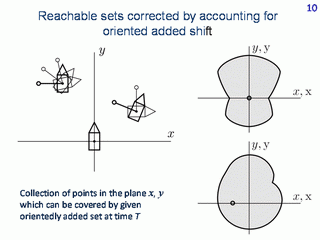Let us fix a set shifted with respect to the origin and attach it rigidly to the car's body. We call it the oriented shift. Take this shifted set as a target for the reference system. The corresponding solvability set for such a target set consists of the reachable set constructed from zero in the plane of the original geometrical coordinates and corrected by accounting for the oriented added set rigidly attached to the car.

## Reachable sets for oriented shift (-1.2, 0)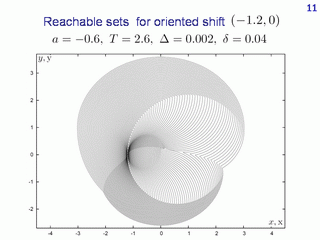Here, we see a very small circle shifted horizontally to the left with respect to the origin. The given-time reachable sets corrected by accounting for the oriented added set are shown. In this case, the computed sets grow in all directions. Therefore they coincide with the corrected time-limited reachable sets. The value of the parameter a is -0.6.

## Reachable sets for oriented shift (-0.5, -0.5)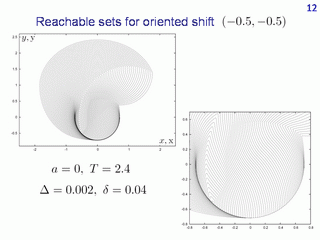On this slide, some other oriented shift with respest to the origin is considered. Parameter a = 0. Pay attention to the thick black curve. When the sets propagate, they bend it. As in the previous example, the corrected given-time reachable sets coincide with the corrected time-limited reachable sets. Every drawn set of the collection corresponds to certain time. The obtained dependence is called the value function.

## Time-limited reachable sets for oriented shift (-0.5, -0.5)The same computation as in the previous slide but for the value of parameter a equal to 0.8 is shown. That is, we decrease the range of changing the possible linear velocity. The corrected time-limited reachable sets are depicted. In the case considered, they do not coincide with the corrected given-time reachable sets.

## Value function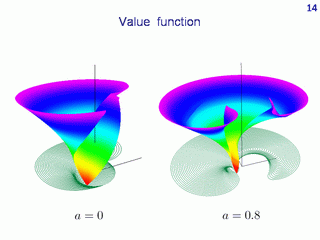Here, graphs of the value function for two last examples are presented. In both examples, the value function is discontinuous.

## Three-dimensional given-time reachable set for Dubins' carThe reachable sets discussed before were reachable sets in the plane of the geometric coordinates of the car. Now, let us go to three-dimensional reachable sets. The third coordinate is the angle θ specifying the current direction of the velocity vector.

Three-dimensional reachable sets are only computed for Dubins' car, which means that the linear velocity magnitude is constant. The computation is performed for the given-time reachable sets only.

Two snapshorts correspoding to the instant π are presented. The colours mark the parts of the boundary with the same type of switching the control u. For example, the red surface is formed using controls of the type 1, -1, 1. (It is proved for Dubins' car that every boundary point of the reachable set can be reached using a piecewise constant control u with two switches at most.)

## Time development of reachable sets for Dubins' car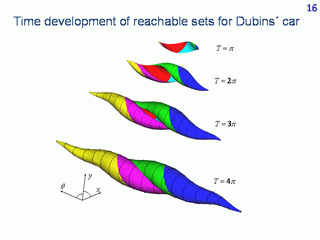Here, the time development of the given-time reachable sets is shown. For small times, the reachable set is similar to a curved leaf. It becomes like a snail with increasing time.

## Violation of simple connectedness of reachable set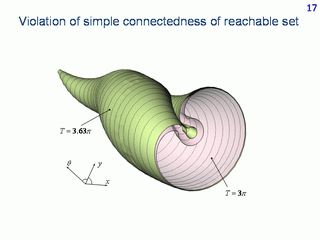In this picture, the reachable sets for two instants are cut by the central plane which is parallel to the plane of geometrical coordinates x,y. The drawing shows the violation of simple connectedness of the three-dimensional reachable set.

## Given-time reachable sets for angle θ modulo 2π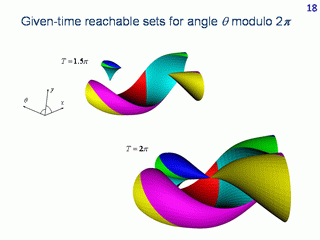It is natural to consider the angle coordinate as being calculated modulo 2π. The sets obtained in this way are shown for the instants 1.5π and 2π.

## Reachable sets in cylindrical coordinates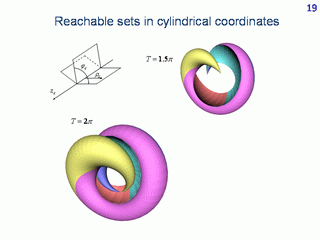If one uses cylindrical coordinates instead of Cartesian ones, the following reachable sets are obtained.

## Authors' works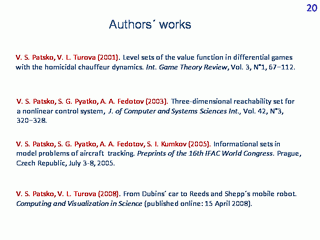Several related works by the authors are presented.

V.S.Patsko
Institute of Mathematics and Mechanics
Ekaterinburg, Russia
patsko@imm.uran.ru

V.L.Turova
Zentrum Mathematik, Technische Universität München
Garching, Germany
turova@ma.tum.de